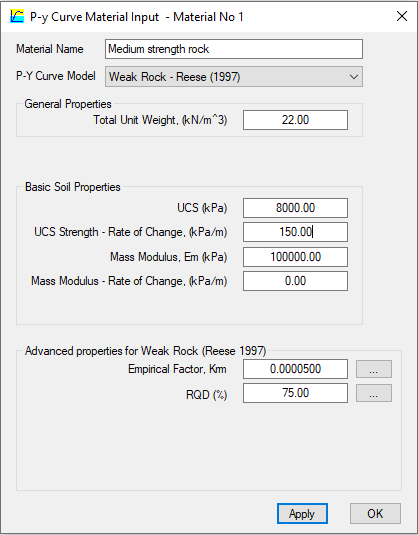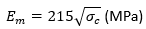top of page

# P-y curve model of weak rock - Reese (1997)

P-Y curves for weak rock in the PileLAT program are calculated using the method proposed by Reese (1997) and shown in the figure below.The ultimate resistance of weak rock is determined according to the following equations:where Pur is ultimate soil resistance per unit length; Alpha-r is the strength reduction factor; Qur is the unconfined compressive strength of rock; D = pile diameter and Xr = depth below rock surface.

The lateral resistance-deflection (P-Y) relationship for weak rock is represented by a three-segment curve. The relationship is described by:YA can be found by solving the following equation:The initial modulus / slope of the P-Y curve, Mir can be determined by:where Em is the rock mass modulus and kir is a dimensionless factor calculated by:The parameter Ym can be determined by:where k-rm is a dimensionless constant and normally ranges from 0.0005 to 0.00005 in the analysis.

In the PileLAT program, the required input parameters for p-y curve of weak rock are shown in the figure below:Total Unit Weight - the total unit weight of the soil material

UCS - unconfined compressive strength (kPa)

UCS Strength – Rate of change - the increase rate of the unconfined compressive strength (UCS) with the depth from the top of the soil layer

Empirical Factor (k-rm) - Dimensionless constant ranging from 0.00005 to 0.0005 and the following dialog can help the users to select this parameter:Mass Modulus, Em - The default value of Em is determined by the method of Rowe and Armitage (1984) as below:where Sigma-c is the unconfined compressive strength of rock.

RQD - this is rock quality designation parameter and varies between 0 and 100%. The following dialog can help the users to select this parameter based on the defect spacing:Mass Modulus – Rate of Change, Em-inc - this is the rock mass modulus increment rate with the layer depth from the layer top.

A typical plot dialog for -y curves of weak rock from the PileLAT program is shown as below:1,412 views